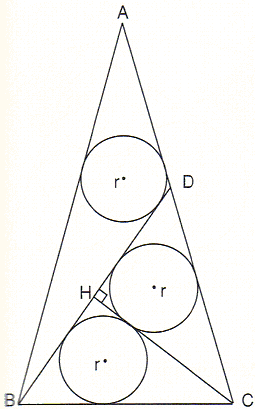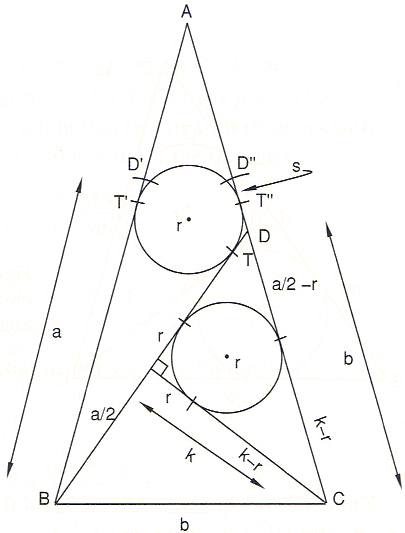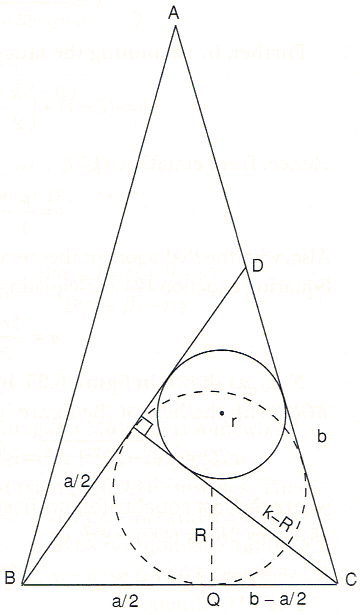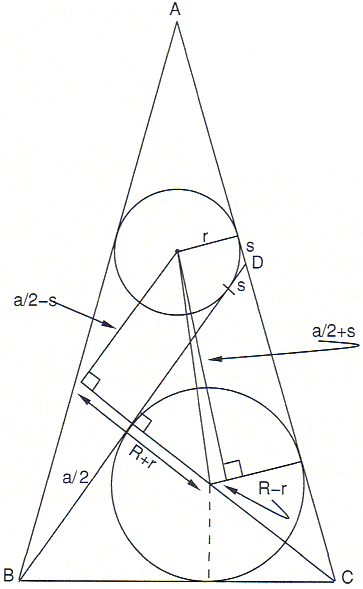# A Restored Sangaku Problem

The sangaku discussed below was originally hung [Fukagawa and Rothman, pp. 194-195] in 1806 in the Atsuta shrine by Ehara Masanori, a disciple of Kusaka Makoto (1764-1833). The tablet, which contained only this problem and no solution, was subsequently lost. However, at an unknown date the mathematician Kitagawa Moko (1763-1839) visited the shrine and recorded the sangaku in his note "Kyuka Sankei," or "Nine Flowers Mathematics," along with his solution. More recently the shrine constructed a replica from Kitagawa's manuscript. Fukagawa and Rothman reproduce Kitagawa's solution with a warning: This may be the most involved exploitation of the Pythagorean theorem you have ever seen.The problem is this: ΔABC is isosceles. Two lines BD and CH divide it into three smaller triangles, each of which circumscribes a circle of the same radius r. Find r in terms of CH.

Following [Fukagawa and Rothman, pp. 212-216], I shall give Kitagawa's solution and then, on a separate page, a solution by J. M. Unger.First, notice from the figure above that triangle CDB is isosceles. Therefore let b = BC = CD, as indicated. We also let a = BD and k = CH. Next, as shown below, mark with a compass two points D' on AB and D'' on AC such that BD = BD' = CD'' = a. Because ΔABC is isosceles, D'T' = D''T'' and by construction D'T' = DT; consequently DT = D''T''.T and T'' are equidistant from D and therefore DT'' = D''T''. Defining s = DT = DT'' we can write

 (1) s = (CD'' - CD) / 2 = (a - b) / 2.

Further, by examining the tangents in the figure above, we see that

 (2) b = (k - r) + (a/2 - r) = a/2 + k - 2r.

Hence, from equation (1)

 (3) s = a / 4 - (k - 2r) / 2.

Also, with the Pythagorean theorem, from either ΔBCH or ΔCDH, b² = (a/2)² + k². Squaring equation (2) and equating the two expressions quickly yields

 (4) a = 4r(k - r) / (k - 2r).

Next, as shown below, inscribe a circle of radius R in triangle BCD.With the help of the figure it is easy to show that

 (5) CQ = √(k - R)² - R² = √k² - 2Rk = b - a/2 = k - 2r,

where the last equality follows from equation (2). Squaring equation (5) gives 2Rk = (4kr) - 4r², or

 (6) R = 2r (k - r) / k.

The strategy is now to eliminate R in favor of r and k. Applying the Pythagorean theorem directly to the figure below gives

(R + r)² + (a/2 - s)² = (R - r)² + (s + a/2)²,or, after simplifying,

 (7) 2Rr = as.

The problem is almost solved. We now replace R on the left of equation (7) by equation (6) and as on the right by the expressions in equations (3) and (4) to get

4r²(k - r) / k = 4r(k - r)/(k - 2r) × [(r(k - r)/(k - 2r) - (k - 2r)/2],

which gives the cubic

k³ - 4rk² - 2kr² + 8r³ = (k² - 2r²)(k - 4r) = 0.

From any of the figures, the relevant root is k = 4r, and thus we have the final result

r = CH / 4.

### References

1. H. Fukagawa, A. Rothman, Sacred Mathematics: Japanese Temple Geometry, Princeton University Press, 2008## Sangaku